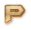# C Arithmetic Operators Online Test

Instructions:
• Total number of questions : 10.
• Time alloted : 10 minutes.
• Each question carry 1 mark.
• No Negative marks
• DO NOT refresh the page.
• All the best :-)

### What You Will Learn in

This C Arithmetic Operators Online Test helps you to Boost your Knowledge in C. In this test, we will cover the topics in the form of questions like

• % is the modulus operator which give the remainder value.
• C language work on the order of precedence of the operator to solve the arithmetic equations.
• We cannot use the floating type variable while doing the % modulus operator.
• Order of precedence of the operator is used to solve the arithmetic equations C language.
• ! is not allowed before equal operator.